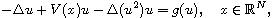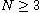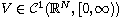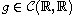Electron. J. Differential Equations, Vol. 2018 (2018), No. 157, pp. 1-13.

### Existence of ground state solutions for quasilinear Schrodinger equations with variable potentials and almost necessary nonlinearities Sitong Chen, Xianhua Tang

Abstract:
In this article we prove the existence of ground state solutions for the quasilinear Schrodinger equationwhere,satisfies mild decay conditions andsatisfies Berestycki-Lions conditions which are almost necessary. In particular, we introduce some new inequalities and techniques to overcome the lack of compactness.

Submitted March 27, 2018. Published August 29, 2018.
Math Subject Classifications: 35J20, 35Q55.
Key Words: Quasilinear Schrodinger equation; ground state solution; Berestycki-Lions conditions.

Show me the PDF file (284 KB), TEX file for this article.Sitong Chen School of Mathematics and Statistics Central South University Changsha, 410083 Hunan, China email: mathsitongchen@163.com Xianhua Tang School of Mathematics and Statistics Central South University Changsha, 410083 Hunan, China email: tangxh@mail.csu.edu.cn